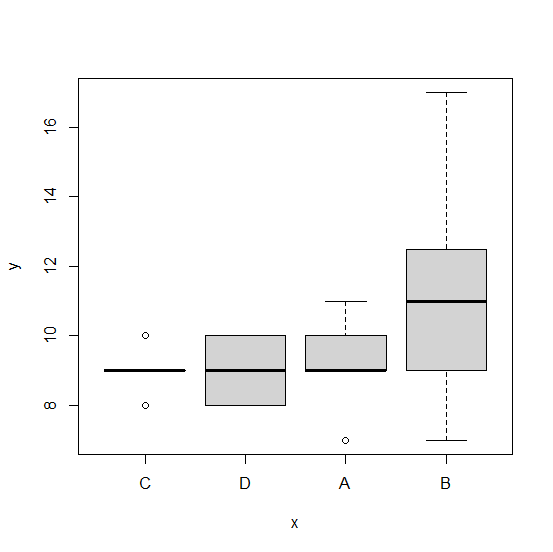# How to create the boxplots in base R ordered by means?

To create the boxplots in base R ordered by means, we first need to order the categorical column based on the mean of the numerical column and then the boxplot will be created.

For example, if we have a data frame df that has a categorical column x and a numerical column y then the boxplot ordered by means can be created by using df$x<- with(df,reorder(x,y,mean)) and boxplot(y~x,data=df). ## Example Consider the below data frame − Live Demo x<-sample(LETTERS[1:4],25,replace=TRUE) y<-rpois(25,10) df<-data.frame(x,y) df ## Output  x y 1 B 7 2 A 7 3 C 8 4 B 8 5 C 9 6 C 9 7 A 10 8 D 10 9 B 11 10 A 9 11 C 10 12 B 10 13 B 13 14 D 10 15 B 10 16 A 9 17 B 12 18 B 8 19 B 12 20 D 8 21 C 9 22 A 11 23 D 8 24 B 17 25 B 13 Ordering the x by mean of y − ## Example df$x<-with(df,reorder(x,y,mean))

Creating the boxplots −

## Example

boxplot(y~x,data=df)

## Output